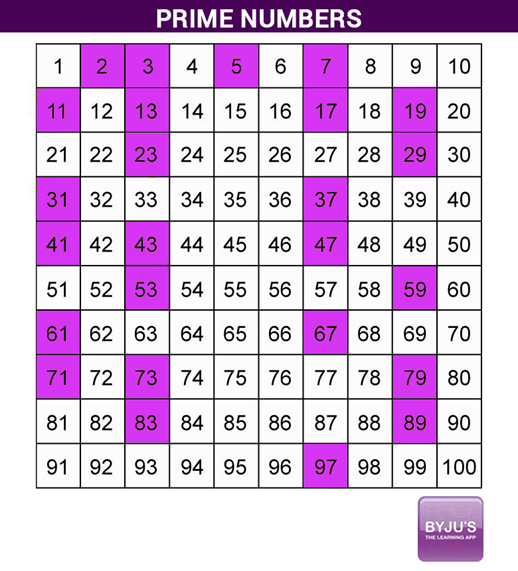# Is 83 a Prime Number

Is 83 a prime number? Before answering this question, let’s understand what a prime number is and how to identify whether a number is prime or not. In mathematics, we come across different types of numbers such as even numbers, odd numbers, rational numbers, whole numbers, etc., As we know, even numbers are divisible by 2, whereas odd numbers leave a remainder as 1 when divided by 2.

Similarly, there are numbers, having exactly two factors 1 and the number itself. Such numbers are 2, 3, 5, 7, 11, etc. These numbers are prime numbers. Mathematically, the numbers other than 1 whose only factors are 1 and the number itself are called Prime numbers. In contrast, numbers having more than two factors are called Composite numbers. For example, the numbers 4, 6, 8, 10, 12, etc are composite. However, 1 is neither a prime nor a composite number.

 Also, check:

## Is 83 Prime or Composite?

In the previous section, you have learned what is a prime and composite number. Now the question is whether the number 83 is prime or not. The answer is, Yes, 83 is a prime number. By the definition of prime numbers, if a number has only 2 factors, i.e. 1 and the number itself, then it is a prime number. If the number has more than 2 factors, then it is called a composite number. However, the factors of 83 are 1 and 83.

### How is 83 a Prime Number?

To explain how 83 becomes a prime number, one should find the factors of 83. As we know, the factors of any number can be found either by the prime factorisation method or the division method. In both cases, we get a pair of numbers that get multiplied and produce the result as the original number. Thus, the pairs of numbers, which produce 83 on multiplication are given as:

1 × 83 = 83

Or

83 × 1 = 83

Here, the number 83 has only two unique numbers that produce the result as 83 on multiplication. Also, these two numbers are 1 and the number itself, i.e. 83.

Therefore, 83 is called a prime number.

However, we can also get the factors by dividing 83 with suitable numbers such as:

83 ÷ 1 = 83

83 ÷ 2 = 42.5 {not a factor}

83 ÷ 3 = 27.667 {not a factor}

….

83 ÷ 83 = 1

Thus, only 1 and 83 divides 83 evenly. Hence, they are the factors of 83.

Also, get the related questions on classification of the number 83 in the table given below:

 Is 83 a prime number? Yes Is 83 a composite number? No What are the factors for 83? 1 and 83 What is (are) prime factors of 83? 83 only Is 83 a perfect square? No Is 83 a perfect cube number? No Is 83 an odd or even number? Odd number What can divide 83 evenly? The factors of 83 divide it evenly, i.e. 1 and 83

Go through the prime numbers chart below that contains the prime numbers before and after 83 which are less than 100.From the above, one can answer the question, Is 83 a Prime number or Not? However, the answer to the question is 83 a prime number.

Apart from the above specified ways, there are two more methods to check whether 83 is a prime number or not.

Method 1: A prime number can be expressed in either of the form of 6n + 1 or 6n – 1, where n is a natural number except the multiples of prime numbers, i.e. 2, 3, 5, 7, 11.

6(14) + 1 = 84 + 1 = 85 > 83

6(14) – 1 = 84 – 1 = 83

That means, 83 can be expressed in the form 6n – 1, where n is a natural number. Hence, it is a prime number.

Method 2:

To know the prime numbers greater than 40, we can use the formula below:

n2 + n + 41, where n = 0, 1, 2, ….., 39

In the above formula, by substituting n = 6, we get;

(6)2 + 6 + 41 = 36 + 6 + 41 = 83

Thus, we can say that 83 is a prime number.

## Frequently Asked Questions – FAQs

### What is the prime factorization of 83?

The prime factorisation of 83 contains only one number, i.e. 83. As it is a prime number, there will be no other prime factors.

### Why is 83 not a composite number?

A number is said to be a composite number, if it has more than two factors. In the case of 83, it has exactly two factors, they are 1 and 83. Hence, 83 is not a composite number.

### What are the factors of 83?

The factors of 83 are 1 and 83.

### Is 83 a prime number yes or no?

Yes, 83 is a prime number.

### What are prime numbers from 1 to 100?

The list of prime numbers from 1 to 100 include the following.
2, 3, 5, 7, 11, 13, 17, 19, 23, 29, 31, 37, 41, 43, 47, 53, 59, 61, 67, 71, 73, 79, 83, 89, 97